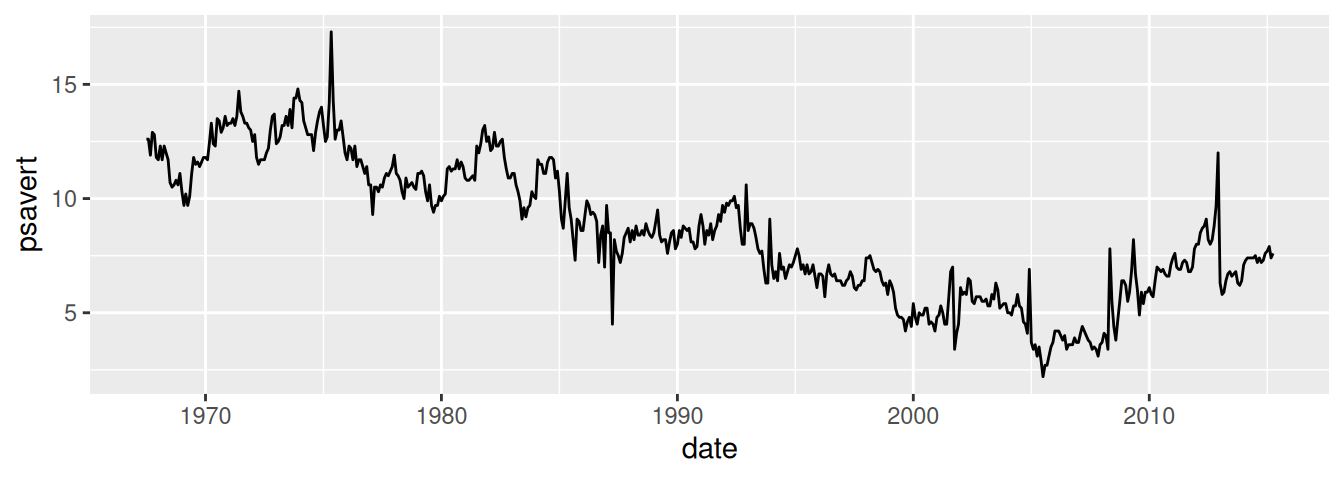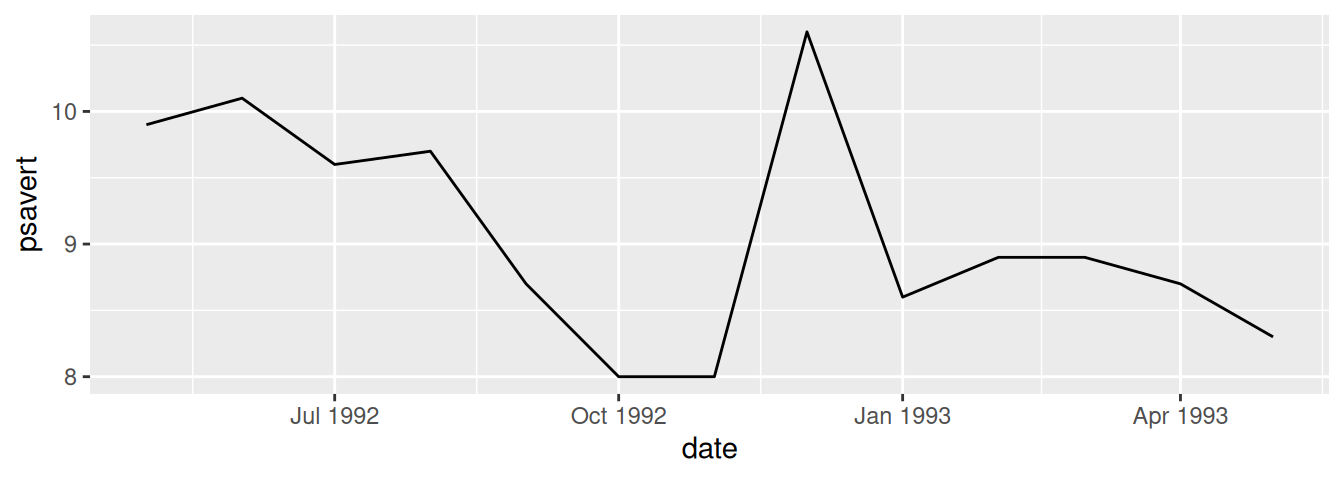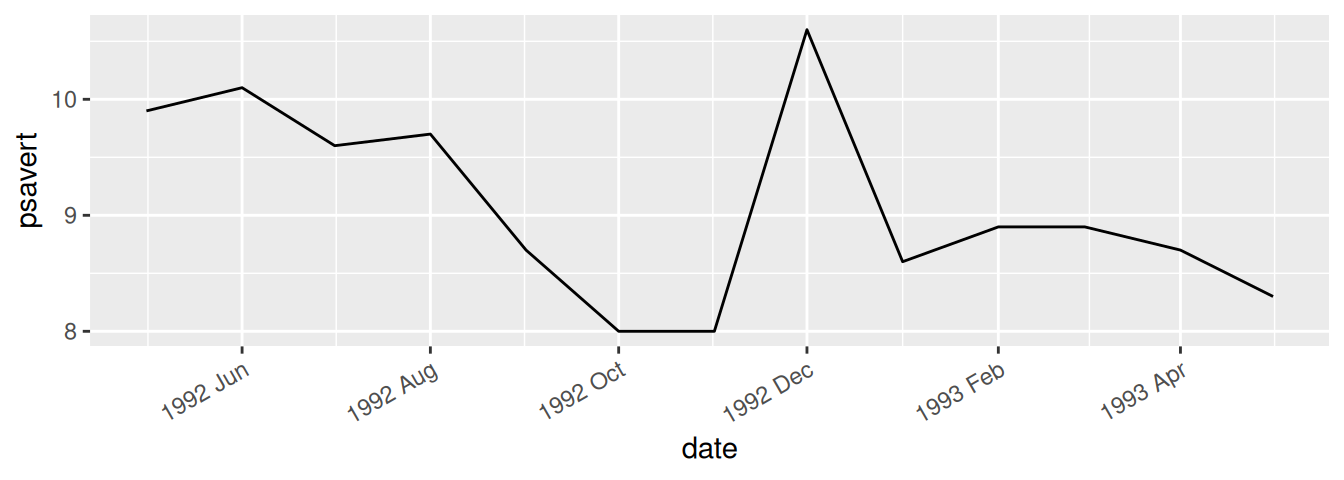## 8.17 Using Dates on an Axis

### 8.17.1 Problem

You want to use dates on an axis.

### 8.17.2 Solution

Map a column of class `Date` to the x- or y-axis. We’ll use the `economics` data set for this example:

``````economics
#> # A tibble: 574 × 6
#>   date         pce    pop psavert uempmed unemploy
#>   <date>     <dbl>  <dbl>   <dbl>   <dbl>    <dbl>
#> 1 1967-07-01  507. 198712    12.6     4.5     2944
#> 2 1967-08-01  510. 198911    12.6     4.7     2945
#> 3 1967-09-01  516. 199113    11.9     4.6     2958
#> 4 1967-10-01  512. 199311    12.9     4.9     3143
#> 5 1967-11-01  517. 199498    12.8     4.7     3066
#> 6 1967-12-01  525. 199657    11.8     4.8     3018
#> # ℹ 568 more rows``````

The column date is an object of class `Date`, and mapping it to x will produce the result shown in Figure 8.37:

``````ggplot(economics, aes(x = date, y = psavert)) +
geom_line()``````Figure 8.37: Dates on the x-axis

### 8.17.3 Discussion

ggplot handles two kinds of time-related objects: dates (objects of class `Date`) and date-times (objects of class `POSIXt`). The difference between these is that `Date` objects represent dates and have a resolution of one day, while `POSIXt` objects represent moments in time and have a resolution of a fraction of a second.

Specifying the breaks is similar to with a numeric axis – the main difference is in specifying the sequence of dates to use. We’ll use a subset of the `economics` data, ranging from mid-1992 to mid-1993. If breaks aren’t specified, they will be automatically selected, as shown in Figure 8.38 (top):

``````library(dplyr)

# Take a subset of economics
econ_mod <- economics %>%
filter(date >= as.Date("1992-05-01") & date <  as.Date("1993-06-01"))

# Create the base plot, which does not specify the breaks
econ_plot <- ggplot(econ_mod, aes(x = date, y = psavert)) +
geom_line()

econ_plot``````

The breaks can be created by using the `seq()` function with starting and ending dates, and an interval (Figure 8.38, bottom):

``````# Specify breaks as a Date vector
datebreaks <- seq(as.Date("1992-06-01"), as.Date("1993-06-01"), by = "2 month")

# Use breaks, and rotate text labels
econ_plot +
scale_x_date(breaks = datebreaks) +
theme(axis.text.x = element_text(angle = 30, hjust = 1))``````Figure 8.38: Top: with default breaks on the x-axis; bottom: with breaks specified

Notice that the formatting of the breaks changed. You can specify the formatting by using the `date_format()` function from the scales package. Here we’ll use `"%Y %b"`, which results in a format like `"1992 Jun"`, as shown in Figure 8.39:

``````library(scales)

econ_plot +
scale_x_date(breaks = datebreaks, labels = date_format("%Y %b")) +
theme(axis.text.x = element_text(angle = 30, hjust = 1))``````Figure 8.39: Line graph with date format specified

Common date format options are shown in Table 8.1. They are to be put in a string that is passed to `date_format()`, and the format specifiers will be replaced with the appropriate values. For example, if you use `"%B %d, %Y"`, it will result in labels like “June 01, 1992”.

Table 8.1: Date format options
Option Description
`%Y` Year with century (2012)
`%y` Year without century (12)
`%m` Month as a decimal number (08)
`%b` Abbreviated month name in current locale (Aug)
`%B` Full month name in current locale (August)
`%d` Day of month as a decimal number (04)
`%U` Week of the year as a decimal number, with Sunday as the first day of the week (00–53)
`%W` Week of the year as a decimal number, with Monday as the first day of the week (00–53)
`%w` Day of week (0–6, Sunday is 0)
`%a` Abbreviated weekday name (Thu)
`%A` Full weekday name (Thursday)

Some of these items are specific to the computer’s locale. Months and days have different names in different languages (the examples here are generated with a US locale). You can change the locale with `Sys.setlocale()`. For example, this will change the date formatting to use an Italian locale:

``````# Mac and Linux
Sys.setlocale("LC_TIME", "it_IT.UTF-8")

# Windows
Sys.setlocale("LC_TIME", "italian")``````

Note that the locale names may differ between platforms, and your computer must have support for the locale installed at the operating system level.

See `?Sys.setlocale` for more about setting the locale.
See `?strptime` for information about converting strings to dates, and for information about formatting the date output.